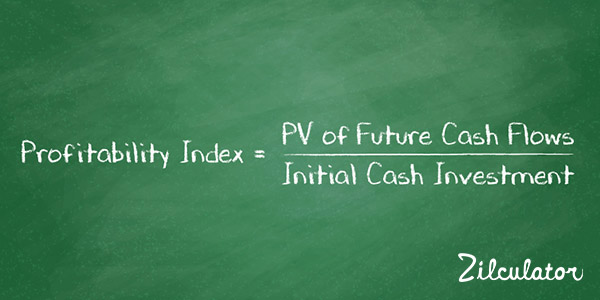# How to Find Profitability Index

## Formula & DefinitionThe profitability index (PI) is similar to the NPV (Net Present Value) method to measure the return on an investment. When calculating NPV, the purchase price is subtracted from the asset's present value (PV) of future cashflow. If the number is zero or a number is positive, you have exceeded or equaled the rate of return that is required (your discount rate).

The profitability index instead calculates the ratio between the initial investment and the present value of future cashflow. If the initial investment and the present value of future cash flow are exactly equal, the index is 1.0. This means that with PI of 1.0 your investment achieves exactly the rate of return you aimed for; or simply that your internal rate of return (IRR) equals your discount rate set in the calculation of present value. If it is above 1.0, you have exceeded the rate of return goal. However, if your ratio is under 1.0, this means that you have not reached the goal and your internal rate of return is lower than your discount rate.

From a glance, it is easy to think that the profitability index is the same as NPV as it also uses present value of future cashflows and the purchase price. However the profitability index makes it easier to compare properties that have a different purchase price. This is because the profitability index is a ratio, showing us each investments proportion for the dollar that is returned vs. the dollar invested initially.

## How to Calculate Profitability Index

1. Calculate present value of all future cash flows using the formula for Discounted Cash Flow.
2. Divide this number by the total initial cash investment using the formula below: